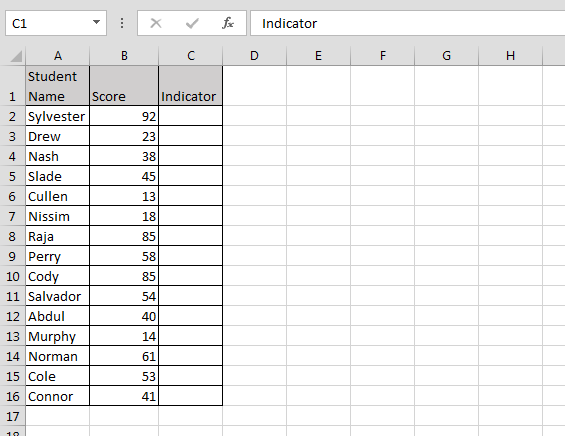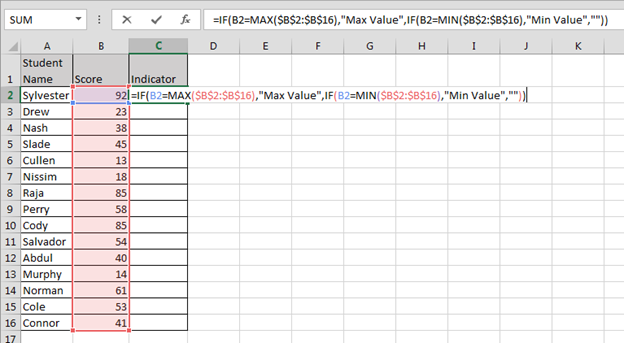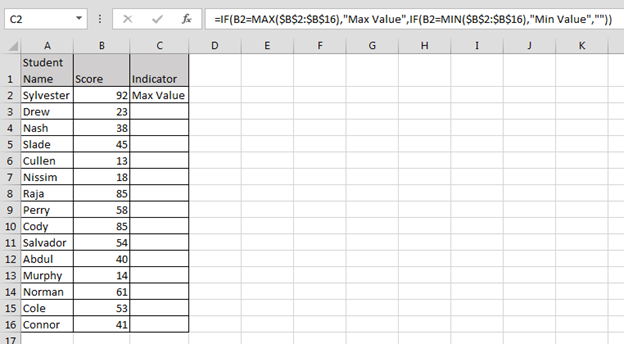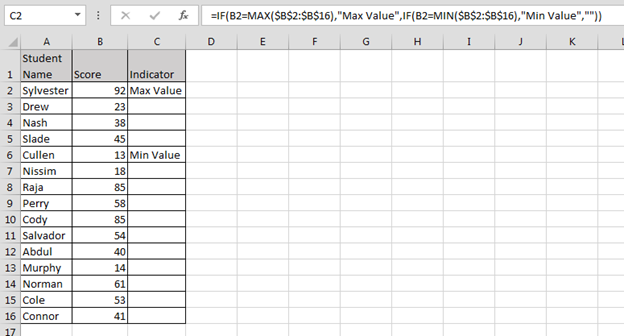# Indicators for the maximal and minimal values

In this article, you’ll learn how to indicate for maximal and minimal values within a range value in Microsoft Excel.

Let’s take an example to understand:-

We have students score data, in which we want to find out minimum and maximum score value.

Problem: - Creating a formula that will indicate the maximum and minimum values in List1
by returning Max Value or Min Value in the cell next to them.• Enter the formula in cell C2
• =IF(B2=MAX(\$B\$2:\$B\$16),"Max Value",IF(B2=MIN(\$B\$2:\$B\$16),"Min Value",""))• Press Enter• Copy the same formula in the rangeIn the above image you can see that we are getting indicator in front of maximum and minimum numbers.

Conclusion: To get the answer we can have multiple conditions in a cell, we don’t need to do it in different ranges.